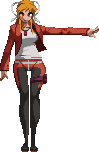# How to add palettes to sffv2 characters (Read 4515 times)

Started by Just No Point, January 25, 2017, 08:25:08 pm
Share this topic:

#### Just No Point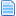##### How to add palettes to sffv2 characters
#1  January 25, 2017, 08:25:08 pm
••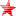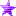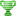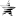For now this only applies to Piccolo but will work with any sffv2 character as long as you know where their palettes are stored.

Open Fighter Factory 3

Load Piccolo and look at his sff. Make sure you have the palettes option enabled for the right hand window.

Scroll through his palettes. In this example his latest palette is 1,94. I'm adding 1,95.

Click the upper left icon to add a new palette as indicated in the image belowIt will ask"Do you want to load the palettes from files?"

Make the palette the next index higher. Since the last he had was 1,94 this one will be 1,95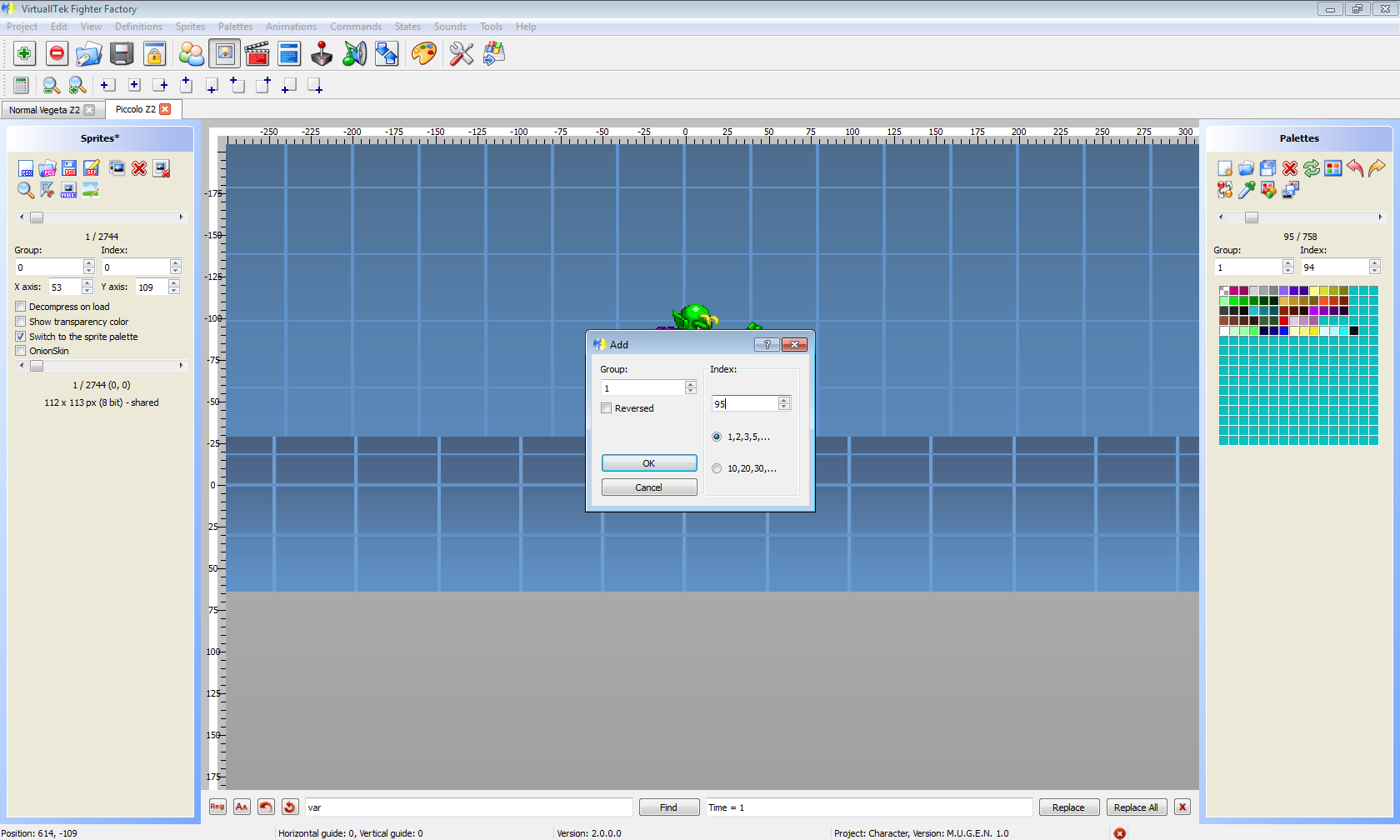That's it!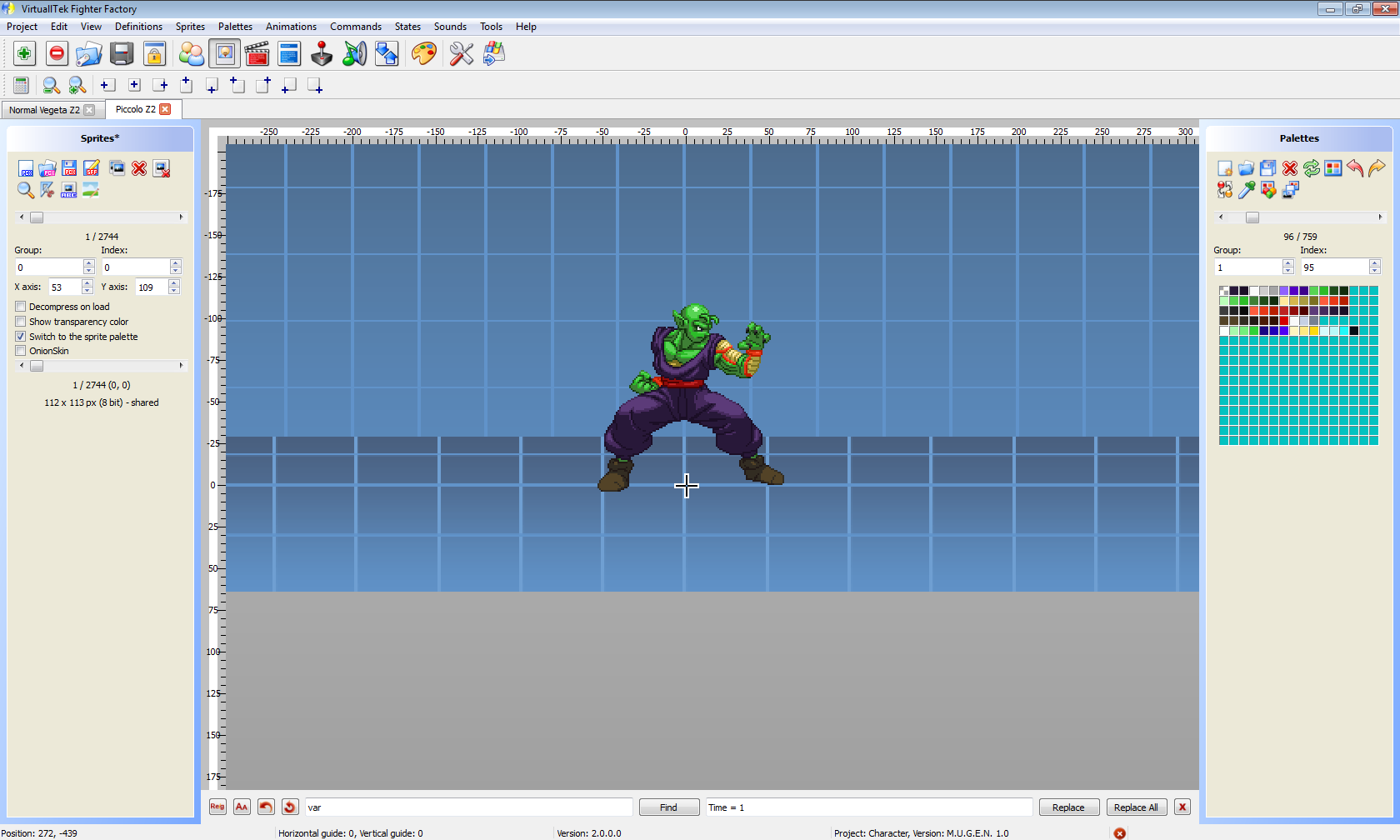Be sure to save the sff!#### Just No Point##### Re: How to add palettes to sffv2 characters
#2  January 25, 2017, 08:25:55 pm
••How to add the new palette to the palette selection option.

Note that currently your build will look a bit different coding wise. Some things have been improved and notes added.

Code:
`;------------------------------;Palette Selection Code[State 5900, Apply Pal]type = RemapPaltrigger1 = var(58)source = 9000,0dest = 1,var(58)[State 0, VarSet]type = VarSettriggerall = var(5) = 1trigger1 = var(58) <= 0trigger2 = var(58) > 95;when you add a palette increase this number by 1var(58) = 1[State 0, VarSet]type = VarSettriggerall = var(5) = 1trigger1 = Facing = -1trigger1 = P2Name = "Piccolo Z2"trigger1 = enemy, var(58) = var(58)var(58) = var(58) + 1[State 0, VarAdd]type = VarAddtriggerall = var(5) = 1triggerall = RoundState = 1triggerall = RoundNo = 1triggerall = !NumExplod(21)trigger1 = Facing = 1trigger1 = command = "fwd"trigger2 = Facing = -1trigger2 = command = "back"var(58) = Cond((P2Name = "Piccolo Z2") && enemy, var(58) = var(58) + 10,11,Cond((Var(58) >= 84), -84,10));You will edit this portion Cond((Var(58) >= ##), -##,10)) increase the ## by 1[State 0, VarAdd]type = VarAddtriggerall = var(5) = 1triggerall = RoundState = 1triggerall = RoundNo = 1triggerall = !NumExplod(21)trigger1 = Facing = 1trigger1 = command = "back"trigger2 = Facing = -1trigger2 = command = "fwd"var(58) = Cond((P2Name = "Piccolo Z2") && enemy, var(58) = var(58) - 10,-11,Cond((Var(58) <= 10), 84,-10));You will edit this portion Cond((Var(58) <= 10), ##,-10) increase the ## by 1[State 0, VarAdd]type = VarAddtriggerall = var(5) = 1triggerall = RoundState = 1triggerall = RoundNo = 1triggerall = !NumExplod(21)trigger1 = command = "up"var(58) = Cond((P2Name = "Piccolo Z2") && enemy, var(58) = var(58) + 1,2,Cond((Var(58) >= 94), -93,1));You will edit this portion Cond((Var(58) >= ##), -##,1)) increase the ## by 1[State 0, VarAdd]type = VarAddtriggerall = var(5) = 1triggerall = RoundState = 1triggerall = RoundNo = 1triggerall = !NumExplod(21)trigger1 = command = "down"var(58) = Cond((P2Name = "Piccolo Z2") && enemy, var(58) = var(58) - 1,-2,Cond((Var(58) <= 1), 93,-1));You will edit this portion Cond((Var(58) <= 1), ##,-1)) increase the ## by 1[State 0, Explod]type = Explodtriggerall = !NumExplod(21)triggerall = var(5) = 1triggerall = RoundState = 1triggerall = RoundNo = 1trigger1 = command = "s"anim = 1606ID = 21removetime = -1[State 0, RemoveExplod]type = RemoveExplodtrigger1 = RoundState = 2id = 21;End of Palette Selection Code;------------------------------`

Basically you just need to increase the ##'s mentioned above by 1

so 84 would become 85
93 would become 94
94 would become 95
example
[State 0, VarSet]
type = VarSet
triggerall = var(5) = 1
trigger1 = var(58) <= 0
trigger2 = var(58) >   94 95;when you add a palette increase this number by 1
var(58) = 1Last Edit: January 25, 2017, 08:56:05 pm by Just No Point

#### AgentofDestiny##### Re: How to add palettes to sffv2 characters
#3  January 27, 2017, 02:02:06 am
••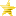• Fate's Persona
• Words hurt more than blades.
Thank you very much for this,it's not as expected
but will try and do.

Even if not,still thank you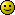~ A swift blade makes monsters tame. ~

#### Just No Point##### Re: How to add palettes to sffv2 characters
#4  January 27, 2017, 02:16:39 am
••If you have questions be sure to ask!